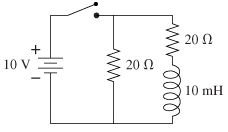# Problem: The switch in the figure has been open for a long time. It is closed at t = 0s.(a) What is the current through the battery immediately after the switch is closed?(b) What is the current through the battery after the switch has been closed a long time?

###### FREE Expert Solution
94% (417 ratings)
###### FREE Expert Solution

$\overline{){\mathbf{V}}{\mathbf{=}}{\mathbf{I}}{\mathbf{R}}}$

Parallel resistors,

$\overline{)\frac{\mathbf{1}}{{\mathbf{R}}_{\mathbf{e}\mathbf{q}}}{\mathbf{=}}\frac{\mathbf{1}}{{\mathbf{R}}_{\mathbf{1}}}{\mathbf{+}}\frac{\mathbf{1}}{{\mathbf{R}}_{\mathbf{2}}}}$

PART A)

The inductor has infinite resistance immediately after the switch is closed. Thus, it acts as an open circuit.

94% (417 ratings)###### Problem Details

The switch in the figure has been open for a long time. It is closed at t = 0s.

(a) What is the current through the battery immediately after the switch is closed?
(b) What is the current through the battery after the switch has been closed a long time?